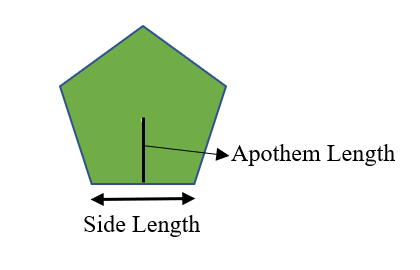# Area of a Pentagon Formula

• Last Updated : 20 Jan, 2022

The concept of the area comes under the topic mensuration which is the branch of geometry that deals with the measurements of shapes i.e., length, area, perimeter, volume etc. for any two-dimensional (2D) or three-dimensional (3D) figures. In this article, we are going to discuss the area of the Pentagon which is a Geometrical 2D figure with 5 sides.

### Area of a Pentagon

The Pentagon area is the region that is enclosed within the 5 sides of a pentagon.The area of the pentagon is calculated by using two approaches. Those are-

1. Using the length of one side.
2. Using Side and Apothem length.

### Area of Pentagon using side length

This approach is used when we had the length of a side of a pentagon. To find the area of the pentagon using the side length the formula is given by,### Area of Pentagon using Side and Apothem length

This approach is used to calculate the area of the pentagon when the side and apothem length of a pentagon is known. The formula of this approach is easy when compared to the above approach formula. It is given by-

Area of Pentagon = (5/2) × (side length) × (Apothem length)

To get more grip on this concept let’s look at a few examples.

### Sample Problems

Problem 1: What is the area of the pentagon with a side of length 5 cm.

Solution:

Given,

Side length (s)= 5cm

Area of Pentagon = (1/4) (√(5(5+2√5))) s2

= (1/4) (√(5(5+2√5))) (5)2

The value for the expression (1/4) (√(5(5+2√5))) is approximately equal to 1.72

= 1.72 × 25

= 43 cm2

The area of pentagon with side 5 cm is 43 cm2.

Problem 2: What is the area of the pentagon with a side of length 6.5 cm.

Solution:

Given,

Side length (s)= 6.5cm

Area of Pentagon = (1/4) (√(5(5+2√5))) s2

= (1/4) (√(5(5+2√5))) (6.5)2

The value for the expression (1/4) (√(5(5+2√5))) is approximately equal to 1.72

= 1.72 × 42.25

= 72.67cm2

The area of pentagon with side 5cm is 72.67cm2.

Problem 3: Find the area of the Pentagon whose length of side and apothem are 5cm,3cm respectively.

Solution:

Given

Side length (s)= 5cm

Apothem length (a)=3cm

Area of pentagon = (5/2) x s x a

= (5/2) x 5 x 3

= 75/2

= 42.5 cm2.

The area of given pentagon is 42.5cm2.

Problem 4: Find the area of the Pentagon whose length of the side is 6cm and apothem length is 5cm?

Solution:

Given

Side length (s)= 6cm

Apothem length (a)=5cm

Area of pentagon = (5/2) x s x a

= (5/2) x 6 x 5

= 150/2

= 75 cm2.

The area of given pentagon is 75cm2.

Problem 5: What is the area of the Pentagon whose length of the side is 4cm and length of apothem is 2 cm.

Solution:

Given

Side length (s)= 4cm

Apothem length (a)=2cm

Area of pentagon = (5/2) x s x a

= (5/2) x 4 x 2

= 20 cm2.

The area of given pentagon with side 4cm and apothem 2 cm is 20 cm2.

My Personal Notes arrow_drop_up